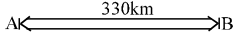## Speed, Time and Distance

#### Speed, Time and Distance

1. Two trains are running in opposite direction with the same speed. If the length of each train is 120 metres and they cross each other in 12 seconds, the speed of each train (in km/hour) is
1. 72
2. 10
3. 36
4. 18

1. Let the speed of each train be x kmph.
Their relative speed
= x + x = 2x kmph

 Time taken = Total length of trains Relative Speed

 = 12 60 × 60

 240 × 1 = 1000 2x

 = 1 = 120 300 1000x

 x = 300 × 120 = 36 1000

The required speed = 36 kmph.

##### Correct Option: C

Let the speed of each train be x kmph.
Their relative speed
= x + x = 2x kmph

 Time taken = Total length of trains Relative Speed

 = 12 60 × 60

 240 × 1 = 1000 2x

 = 1 = 120 300 1000x

 x = 300 × 120 = 36 1000

The required speed = 36 kmph.

1. Two trains, one 160 m and the other 140 m long are running in opposite directions on parallel rails, the first at 77 km an hour and the other at 67 km an hour. How long will they take to cross each other?
1. 7 seconds
2.  7 1 seconds 2
3. 6 seconds
4. 10 seconds

1. If two trains be moving in opposite directions at rate u and v kmph respectively, then their
relative speed = (u + v) kmph.
Further, if their length be x and y km. then time taken to cross

 each other = x + y hours. u + v

Here,
Total length = 160 + 140 = 300m.
Relative speed = (77 + 67) kmph
 = 144 kmph = 144 × 5 m/s or 40 m/sec. 18

 ∴  Time = 300 = 7 1 Seconds 40 2

##### Correct Option: B

If two trains be moving in opposite directions at rate u and v kmph respectively, then their
relative speed = (u + v) kmph.
Further, if their length be x and y km. then time taken to cross

 each other = x + y hours. u + v

Here,
Total length = 160 + 140 = 300m.
Relative speed = (77 + 67) kmph
 = 144 kmph = 144 × 5 m/s or 40 m/sec. 18

 ∴  Time = 300 = 7 1 Seconds 40 2

1. Two men are standing on opposite ends of a bridge 1200 metres long. If they walk towards each other at the rate of 5m/minute and 10m/minute respectively, in how much time will they meet each other ?
1. 60 minutes
2. 80 minutes
3. 85 minutes
4. 90 minutes

1. Men are walking in opposite directions. Hence, they will cover the length of bridge at their relative speed.

 Required time = 1200 = 80 minutes (5 + 10)

##### Correct Option: B

Men are walking in opposite directions. Hence, they will cover the length of bridge at their relative speed.

 Required time = 1200 = 80 minutes (5 + 10)

1. The distance between two cities A and B is 330 km. A train starts from A at 8 a.m. and travels towards B at 60 km/hr. Another train starts from B at 9 a.m. and travels towards A at 75 km/hr. At what time do they meet?
1. 10 a.m.
2. 10 : 30 a.m.
3. 11 a.m.
4. 11 : 30 a.m.

1. Distance travelled by first train in one hour
= 60 × 1 = 60kmTherefore, distance between two train at 9 a.m.
= 330 – 60 = 270 km
Now, Relative speed of two trains
= 60 + 75 = 135 km/hr
∴  Time of meeting of two trains

 = 270 = 2 hrs. 135

Therefore, both the trains will meet at 9 + 2 = 11 A.M.

##### Correct Option: C

Distance travelled by first train in one hour
= 60 × 1 = 60kmTherefore, distance between two train at 9 a.m.
= 330 – 60 = 270 km
Now, Relative speed of two trains
= 60 + 75 = 135 km/hr
∴  Time of meeting of two trains

 = 270 = 2 hrs. 135

Therefore, both the trains will meet at 9 + 2 = 11 A.M.

1. A train 100 metre long is running at a speed of 120 km/hr. The time taken to pass a person standing near the line is
1. 1 second
2. 3 seconds
3. 5 seconds
4. 7 seconds

1. Speed of train = 120 kmph.

 =120 × 5m./sec. 18

 = 100 m./sec. 3

 ∴  Required time = Length of train Speed of train

 =100seconds (100/3)

##### Correct Option: B

Speed of train = 120 kmph.

 =120 × 5m./sec. 18

 = 100 m./sec. 3

 ∴  Required time = Length of train Speed of train

 =100seconds (100/3)